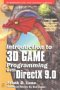# Maths - Inverse Vector

 By: Nobody/Anonymous - nobodyRE: Vector division   2006-01-14 15:28 You are correct, neither dot-product nor cross-product has a general multiplicative inverse, for obvious reasons.    However under the geometric product there is. Here one vector divded by another yield a quaternion that rotates/scales one vector into the other.    a = Q * b  Q = a / b    Or put another way, if we have 2 ratios of vectors    a / b = c / d    this means that a relates to b (in terms of rotation angle and scalar factor) as c relates to d.    The quaternion from vector division a/b is    Q.w = a dot b / b^2  Q.v = a cross b / b^2    However, the direct multiplication Q * a of a quaternion with a vector is only useful if both are on the same axis.    To use Q with an arbitrary vector and the usual sandwich product Q * v * ~Q you need to sqrt() the quaternion.    Q = sqrt( a / b )    a = Q * b * ~Q
 By: Martin Baker - martinbakerRE: Vector division   2006-01-15 10:43 Thanks very much, this is very interesting, I have been trying to work out where the "sandwich product" comes from, it looks like some sort of morphism, but Ive not managed to work it out.    Ill have to work through this in more detail. Would you consider this as a rigorous derivation of the "sandwich product" ?    Martin
 By: Nobody/Anonymous - nobodyRE: Vector division   2006-01-15 11:47 You may note that the sandwich product looks a lot like the matrix similarity transform that was mentioned in the other thread:    M' = F * M * F^-1    where F is the a space conversion an M is to be converted. So it looks like a morphism.    In dynamics, a rotation is generated when two equal but opposite forces act on a body. Symbolically, a quaternion from the left and an opposite one from the right rotate the vector in the middle. I think this is not just a coincidence.    Have you tried this link?  http://modelingnts.la.asu.edu/    lots of material, espc  http://modelingnts.la.asu.edu/html/ComputationalGeometry.html
 By: Martin Baker - martinbakerRE: Vector division   2006-01-16 07:41 Thanks very much there is a lot for me to follow-up here so Ive got a lot of work to do!    Also Ill do some thinking about the interpretation of "quaternion that rotates/scales one vector into the other"    Martin
 By: Martin Baker - martinbakerRE: Vector division   2006-01-16 10:44 I have been trying to check how we can get an algebra where: a = Q * b    Using 3D geometric multiplication as described here:  https://www.euclideanspace.com/maths/algebra/clifford/d3/  I can see that:  vector * vector = bivector + scalar  So if we define the quaternion as bivector + scalar then we have:  vector * vector = quaternion     but we want a/b = Q so if we try  vector * quaternion = vector * (bivector + scalar) = vector + trivector    This trivector seems to spoil things so Ive done something wrong here, I guess its wrong to say quaternion = bivector + scalar, I suspect we need to include its dual somehow?    Ive not had a chance to follow-up the references yet, so Ill try to get an answer from them.    Martin

 metadata block see also: Correspondence about this page Book Shop - Further reading. Where I can, I have put links to Amazon for books that are relevant to the subject, click on the appropriate country flag to get more details of the book or to buy it from them.Introduction to 3D Game Programming with DirectX 9.0 - This is quite a small book but it has good concise information with subjects like, maths introduction and picking.If you are interested in 3D games, this looks like a good book to have on the shelf. If, like me, you want to have know the theory and how it is derived then there is a lot for you here. Including - Graphics pipeline, scenegraph, picking, collision detection, bezier curves, surfaces, key frame animation, level of detail, terrain, quadtrees & octtrees, special effects, numerical methods. Includes CDROM with code. This book contains more mathematically rigorous methods for picking than described above. Other Math Books Specific to this page here:

This site may have errors. Don't use for critical systems.